Ex 12.2

Chapter 12 Class 9 Herons Formula (Term 1)
Serial order wise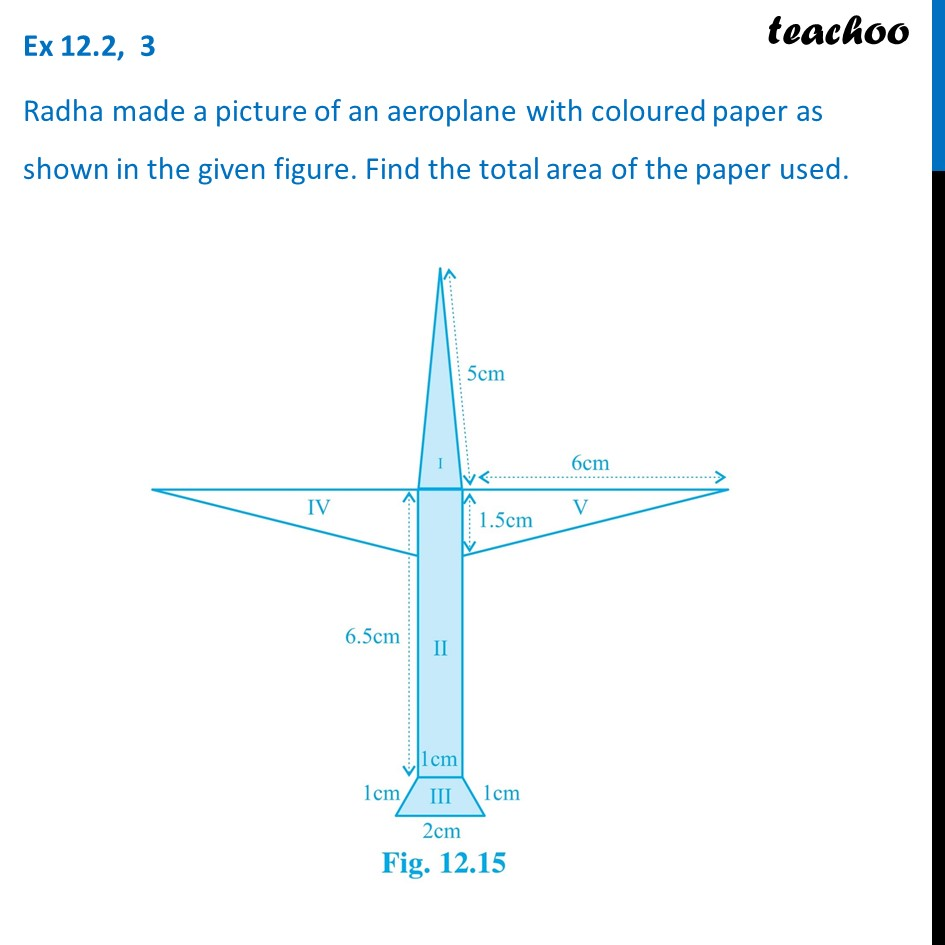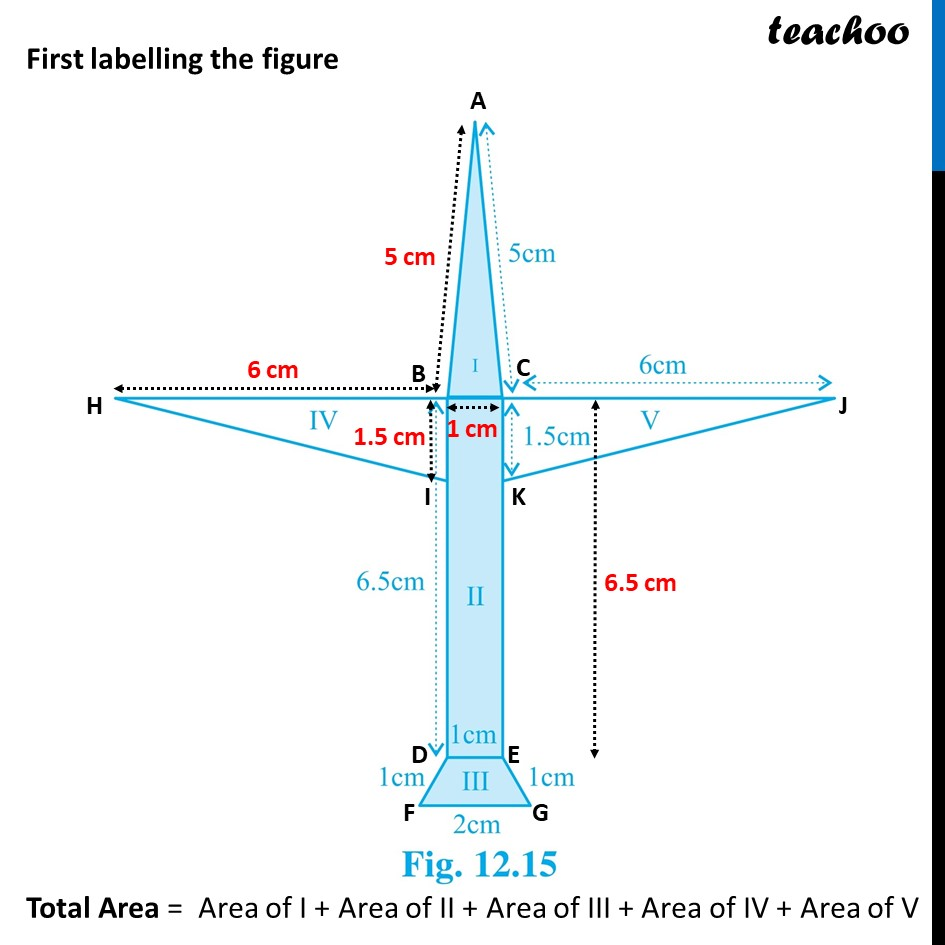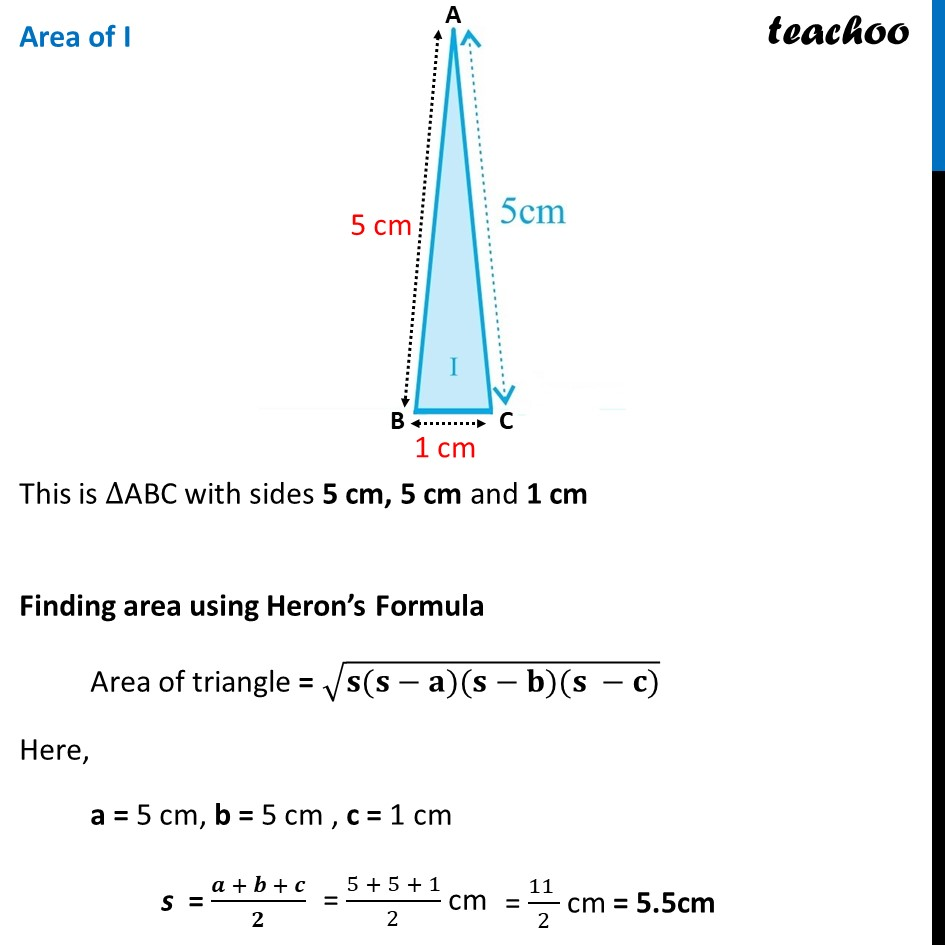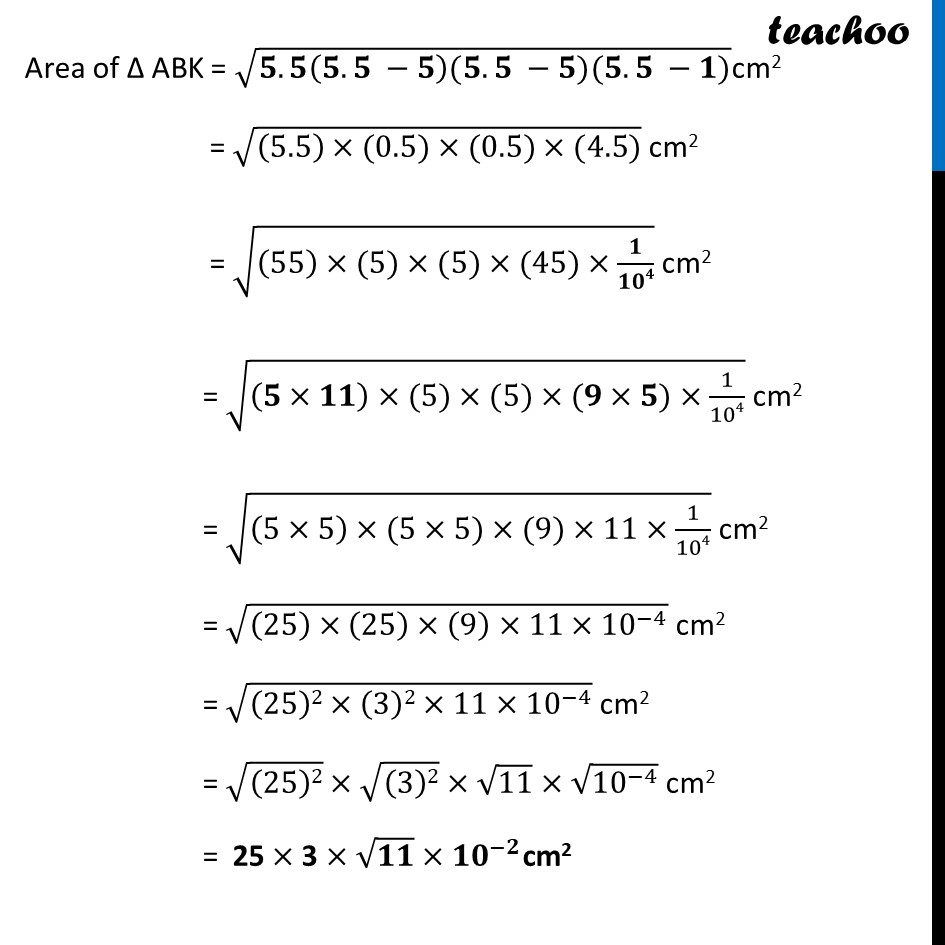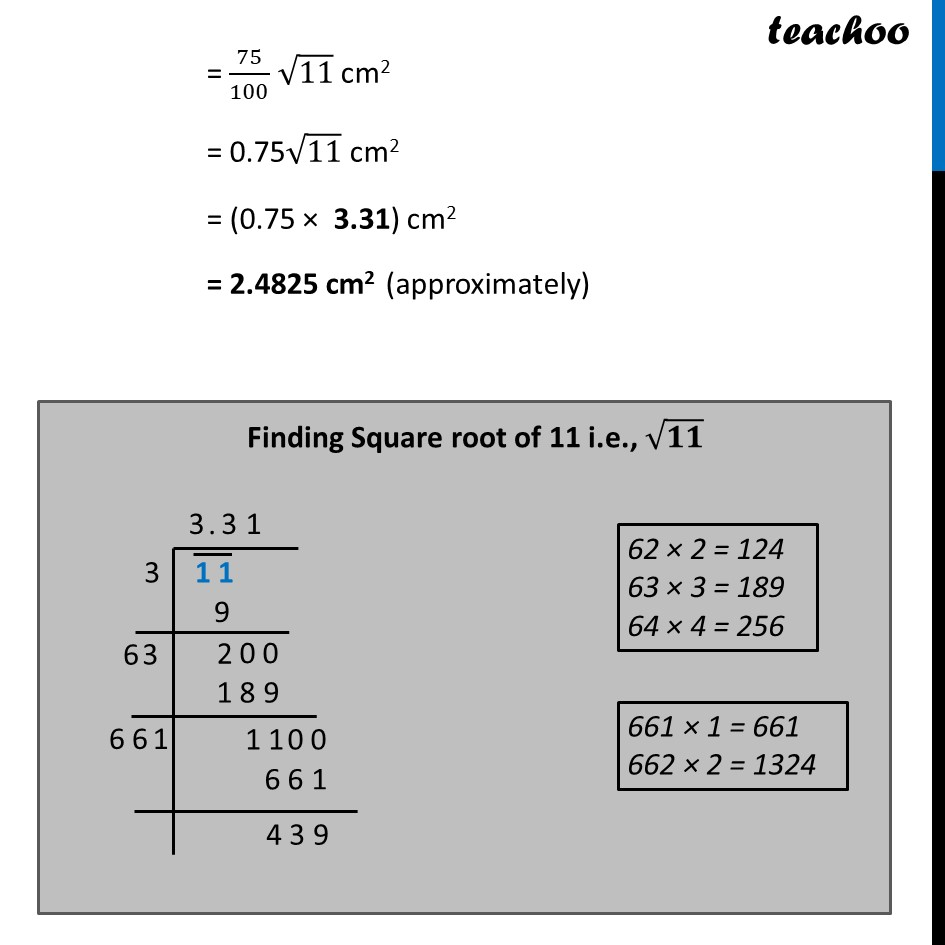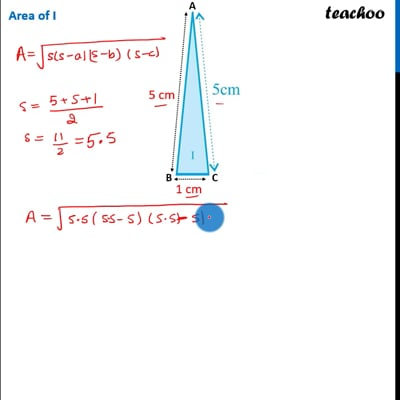This video is only available for Teachoo black users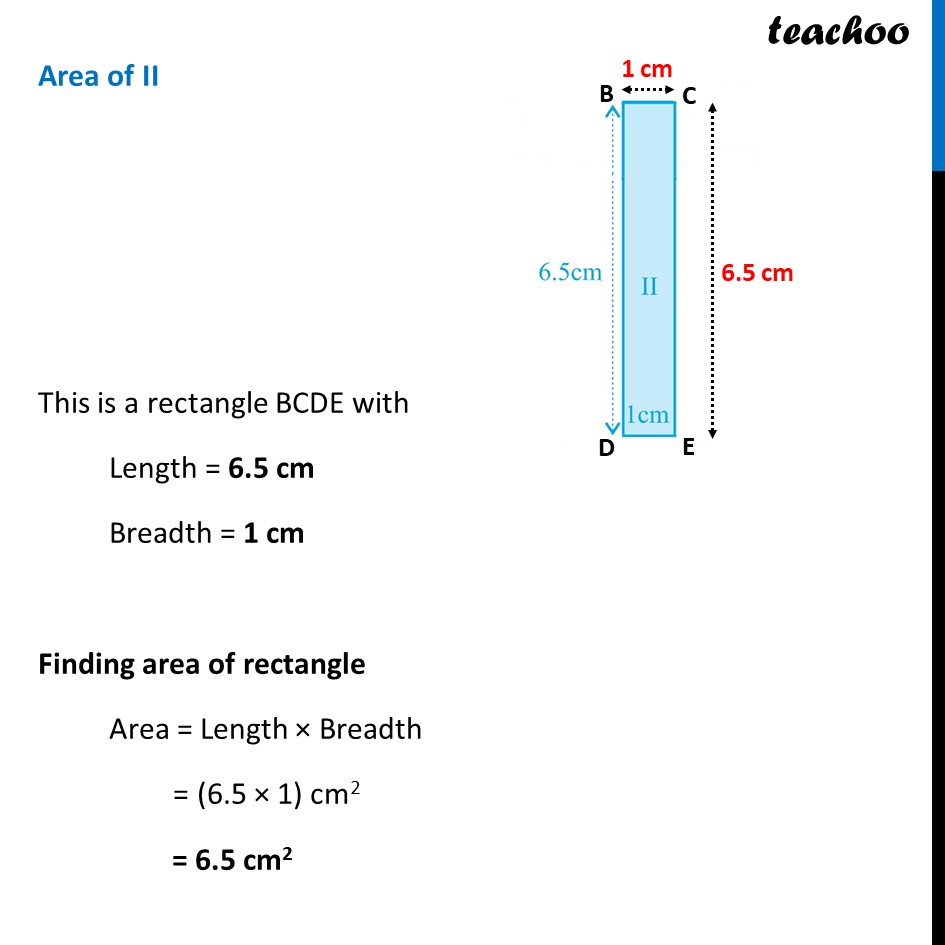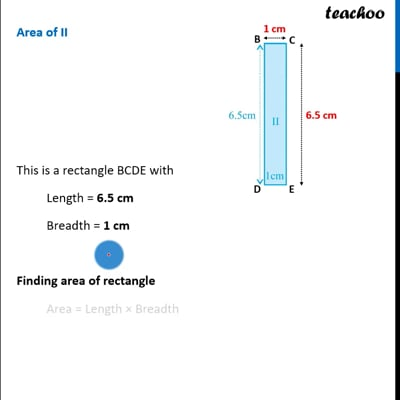This video is only available for Teachoo black users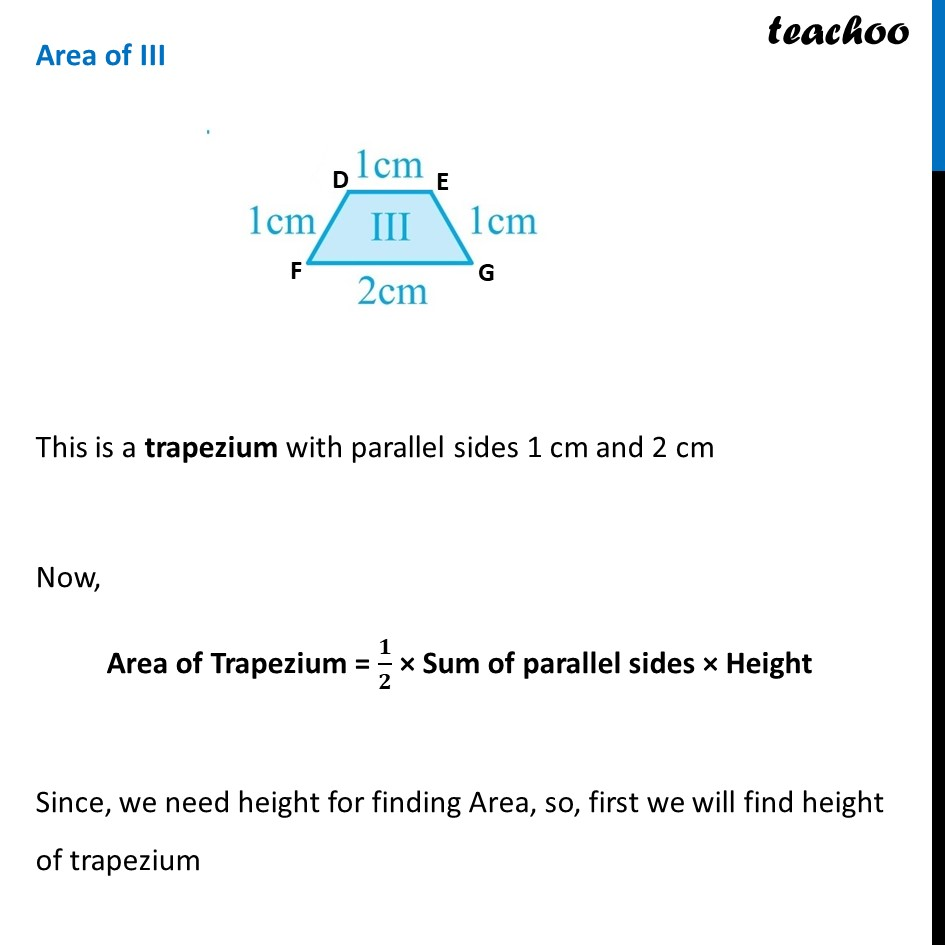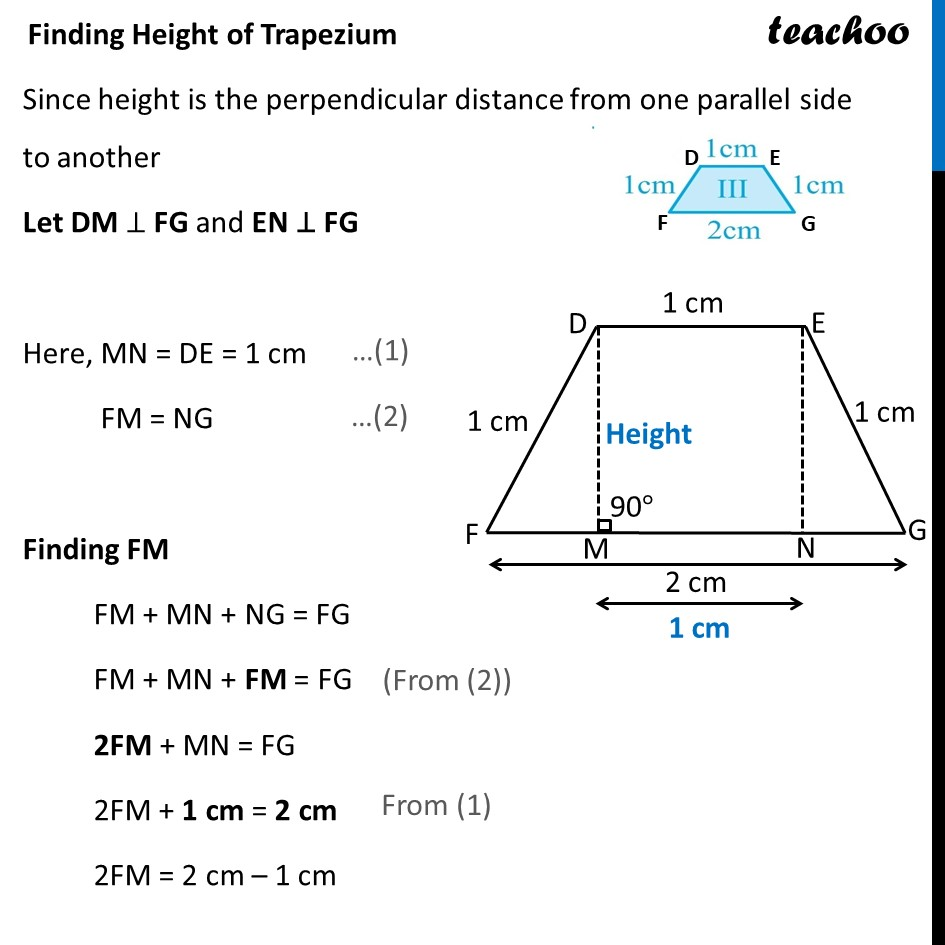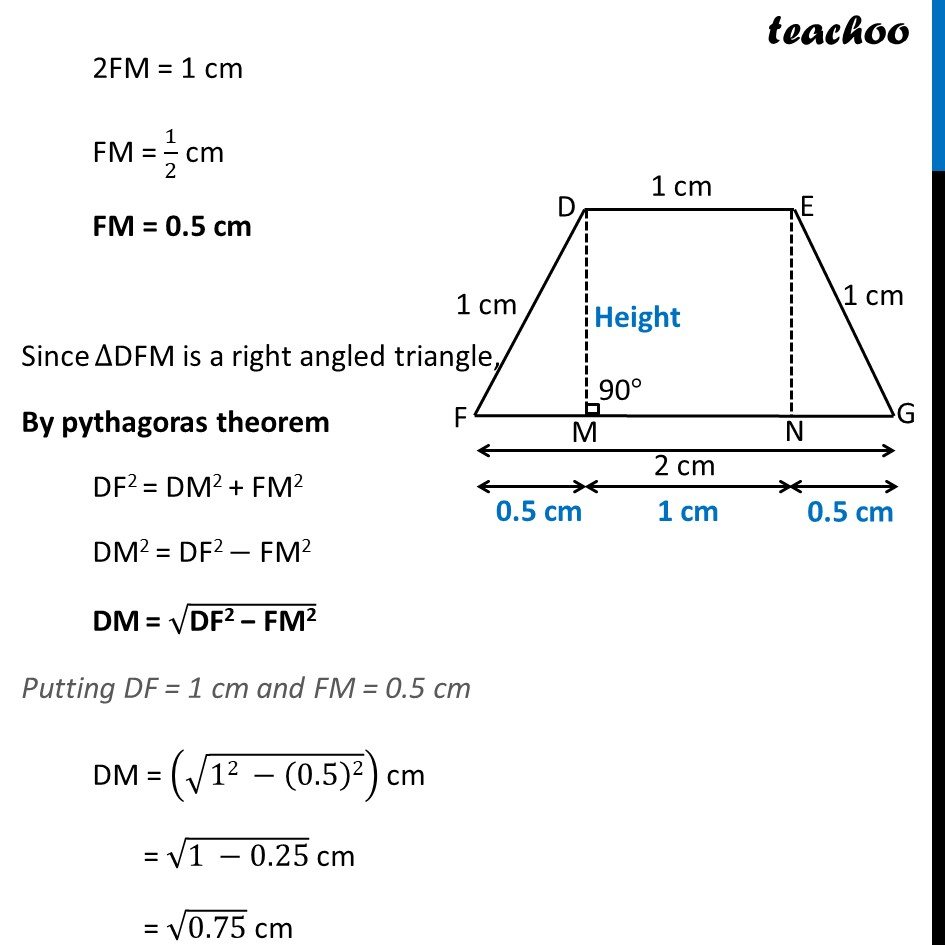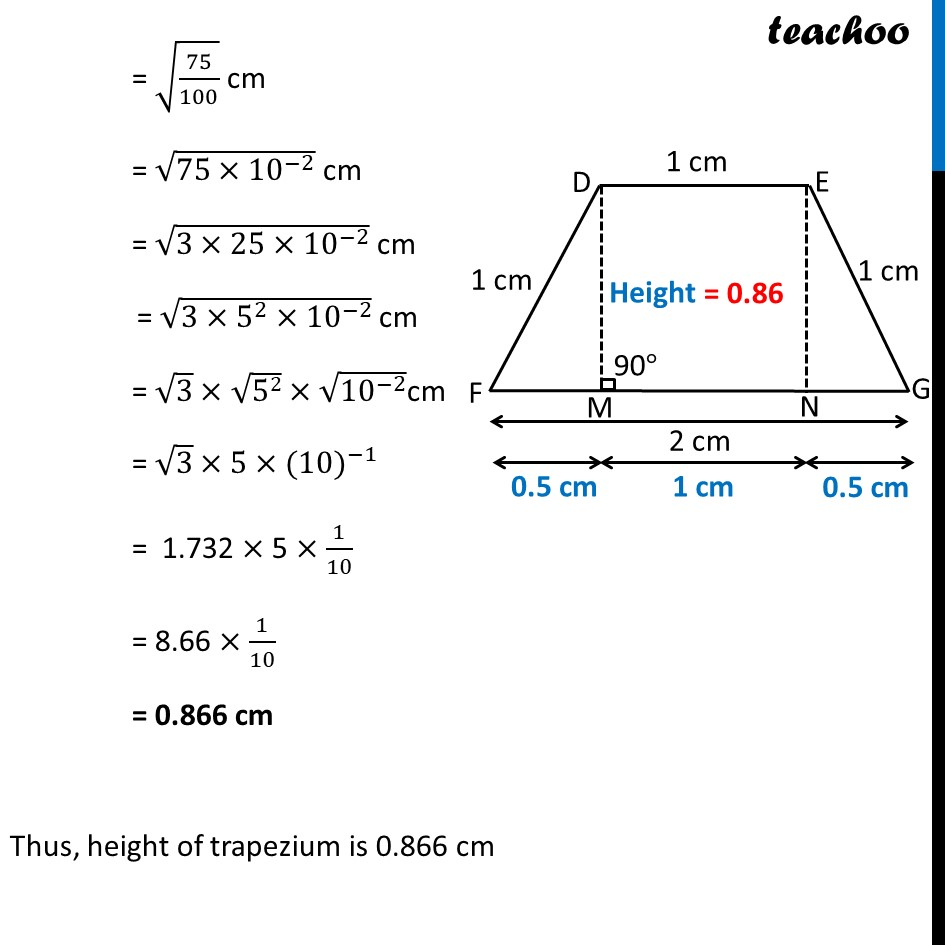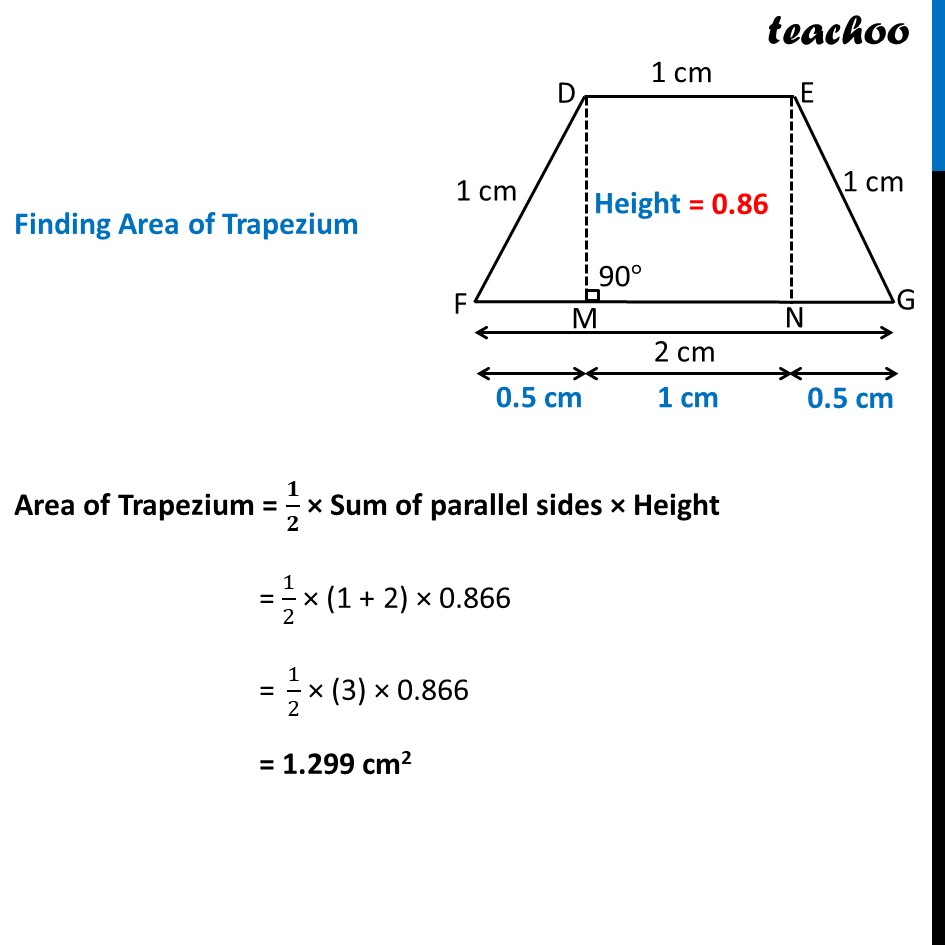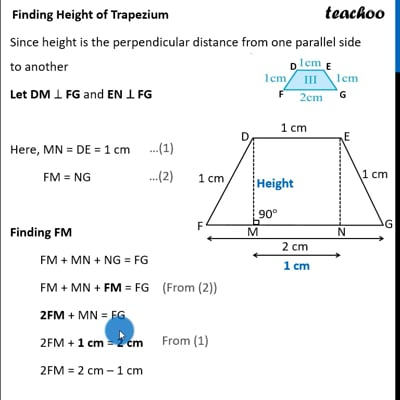This video is only available for Teachoo black users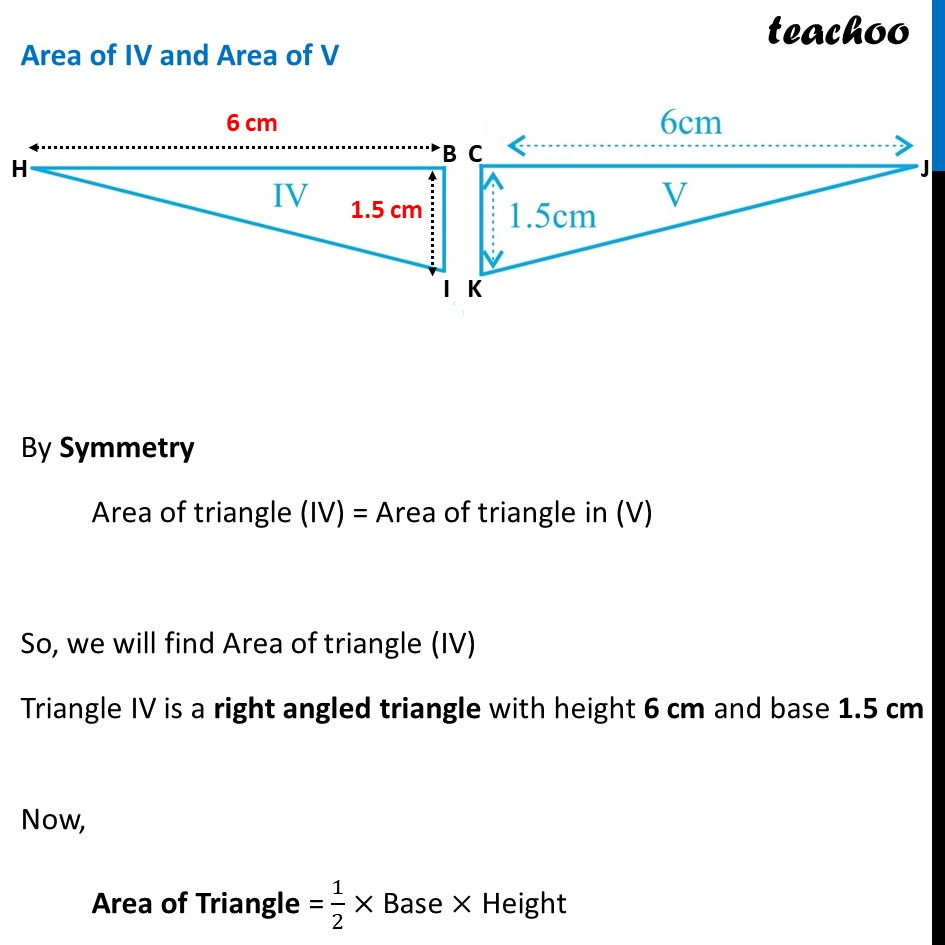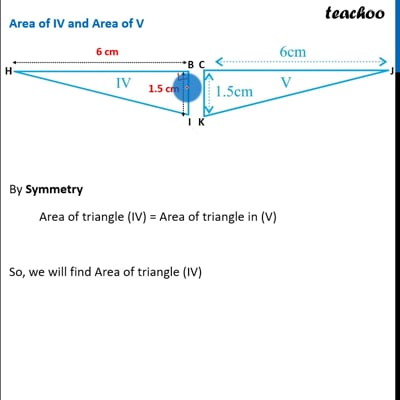This video is only available for Teachoo black users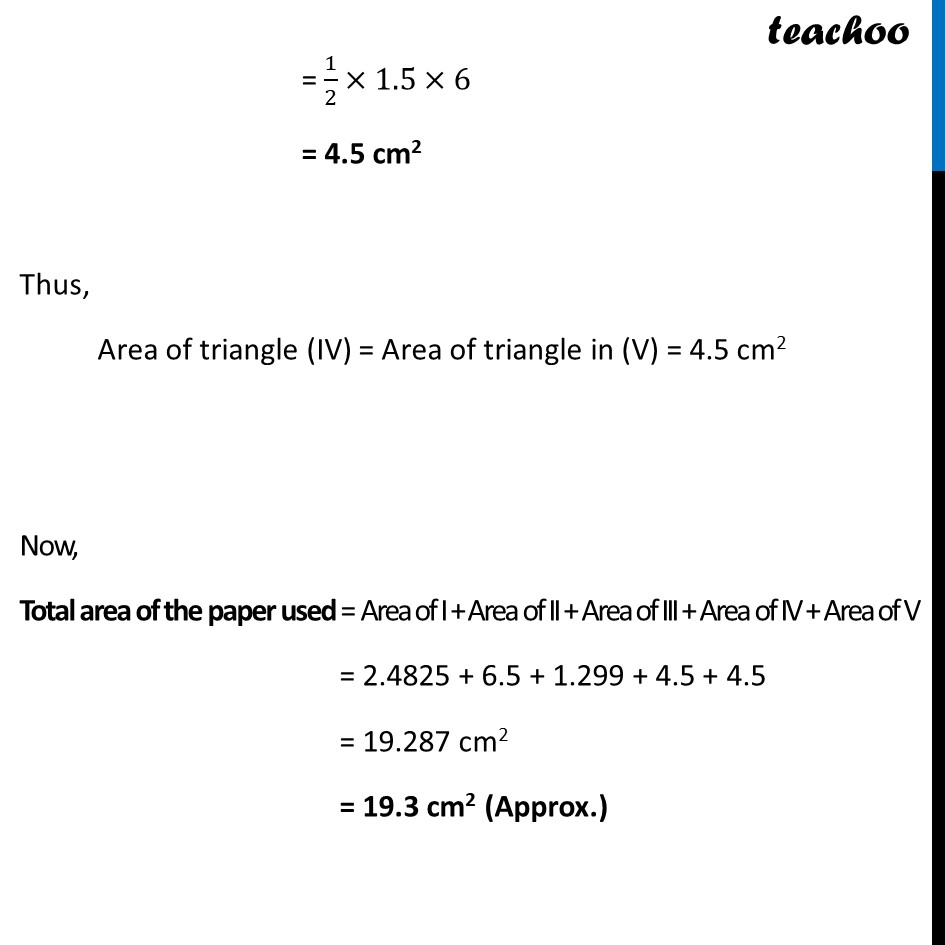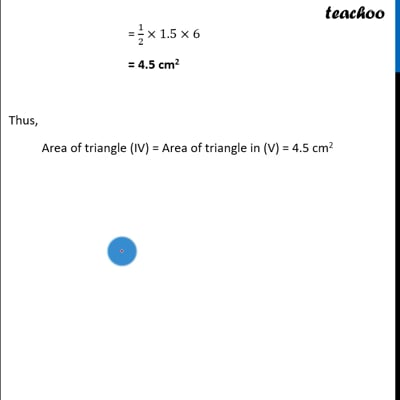This video is only available for Teachoo black users

### Transcript

Ex 12.2, 3 Radha made a picture of an aeroplane with coloured paper as shown in the given figure. Find the total area of the paper used. First labelling the figure Total Area = Area of I + Area of II + Area of III + Area of IV + Area of V Area of I This is ∆ABC with sides 5 cm, 5 cm and 1 cm Finding area using Heron’s Formula Area of triangle = √(𝐬(𝐬−𝐚)(𝐬−𝐛)(𝐬 −𝐜)) Here, a = 5 cm, b = 5 cm , c = 1 cm s = (𝒂 + 𝒃 + 𝒄)/𝟐 Area of Δ ABK = √(𝟓.𝟓(𝟓.𝟓 −𝟓)(𝟓.𝟓 −𝟓)(𝟓.𝟓 −𝟏))cm2 = √((5.5)×(0.5)×(0.5)×(4.5)) cm2 = √((55)×(5)×(5)×(45)×𝟏/𝟏𝟎𝟒) cm2 = √((𝟓×𝟏𝟏)×(5)×(5)×(𝟗×𝟓)×1/104) cm2 = √((5×5)×(5×5)×(9)×11×1/104) cm2 = √((25)×(25)×(9)×11×10^(−4) ) cm2 = √((25)2×(3)2×11×10^(−4) ) cm2 = √((25)2)×√((3)2) ×√11×√(10^(−4) ) cm2 = 25 × 3 × √𝟏𝟏 × 〖𝟏𝟎〗^(−𝟐)cm2 = 75/100 √11 cm2 = 0.75√11 cm2 = (0.75 × 3.31) cm2 = 2.4825 cm2 (approximately) Area of II This is a rectangle BCDE with Length = 6.5 cm Breadth = 1 cm Finding area of rectangle Area = Length × Breadth = (6.5 × 1) cm2 = 6.5 cm2 Area of III This is a trapezium with parallel sides 1 cm and 2 cm Now, Area of Trapezium = 𝟏/𝟐 × Sum of parallel sides × Height Since, we need height for finding Area, so, first we will find height of trapezium Finding Height of Trapezium Since height is the perpendicular distance from one parallel side to another Let DM ⊥ FG and EN ⊥ FG Here, MN = DE = 1 cm FM = NG Finding FM FM + MN + NG = FG FM + MN + FM = FG 2FM + MN = FG 2FM + 1 cm = 2 cm 2FM = 2 cm – 1 cm 2FM = 1 cm FM = 1/2 cm FM = 0.5 cm Since ΔDFM is a right angled triangle, By pythagoras theorem DF2 = DM2 + FM2 DM2 = DF2 − FM2 DM = √("DF2 − FM2" ) Putting DF = 1 cm and FM = 0.5 cm DM = (√(12 −(0.5)2)) cm = √(1 −0.25) cm = √0.75 cm = √(75/100) cm = √(75×10^(−2) ) cm = √(3×25×10^(−2) ) cm = √(3×5^2×10^(−2) ) cm = √3× √52 ×√(10^(−2) )cm = √3×5 × 〖(10)〗^(−1) = 1.732 × 5 ×1/10 = 8.66 ×1/10 = 0.866 cm Thus, height of trapezium is 0.866 cm Finding Area of Trapezium Area of Trapezium = 𝟏/𝟐 × Sum of parallel sides × Height = 1/2 × (1 + 2) × 0.866 = 1/2 × (3) × 0.866 = 1.299 cm2 Area of IV and Area of V By Symmetry Area of triangle (IV) = Area of triangle in (V) So, we will find Area of triangle (IV) Triangle IV is a right angled triangle with height 6 cm and base 1.5 cm Now, Area of Triangle = 1/2 × Base × Height = 1/2×1.5×6 = 4.5 cm2 Thus, Area of triangle (IV) = Area of triangle in (V) = 4.5 cm2 Now, Total area of the paper used = Area of I + Area of II + Area of III + Area of IV + Area of V = 2.4825 + 6.5 + 1.299 + 4.5 + 4.5 = 19.287 cm2 = 19.3 cm2 (Approx.)